# Perturb-and-MAP Random Fields: Reducing Random Sampling to Optimization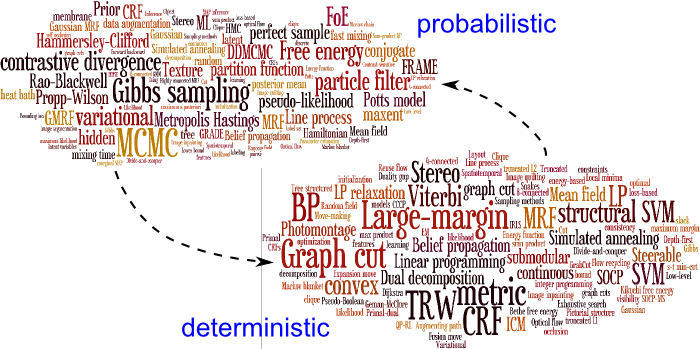Perturb-and-MAP random fields try to bridge the gap between probabilistic and deterministic approaches to MRF modeling.

## Overview

We have been developing a new Perturb-and-MAP framework for random sampling in Markov random fields (MRF). We generate a random sample from the whole field at once by first injecting random noise to the MRF local potentials, then finding the least energy configuration of the perturbed energy function. By properly designing the noise injection process we can generate exact Gibbs samples from Gaussian MRFs and good approximate samples from discrete-label MRFs. With Perturb-and-MAP random fields we thus turn powerful deterministic energy minimization methods into efficient probabilistic random sampling algorithms. By completely avoiding costly MCMC, we can generate in a fraction of a second independent random samples from million-node networks. Applications include model parameter learning and solution uncertainty quantification in computer vision applications.

### Problem statement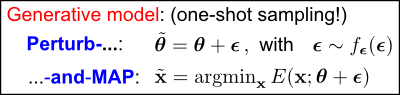We consider a graph with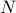nodes which can take either discrete or continuous values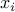. Our starting point is a deterministic energy function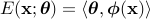, where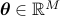is a vector of real parameters and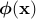is a vector of data-dependent potentials. In the Perturb-and-MAP random field we generate a sample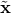by first adding noise to the parameters, followed by finding the most probable (MAP) state of the perturbed energy function, as shown in the box to the left. We can design the perturbation density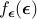so as the resulting model is identical or approximates closely the standard Gibbs MRF whose probability is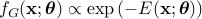. The advantage of the Perturb-and-MAP approach over standard MRF simulation techniques is that it avoids MCMC, instead reducing sampling to a typically easier to solve energy minimization problem.

## Discrete-Label Markov Random Fields (ICCV-11 paper)

In discrete-label MRFs the nodescan take one out of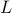possible labels. This class of models, rooted to the classic Ising and Potts models in statistical physics, is widely used in image analysis and computer vision in applications such as image segmentation, stereo, and optical flow estimation. Powerful algorithms such as graph cuts, dual decomposition, and linear programming relaxations can efficiently find or approximate the minimum energy configuration for many discrete-label energy models used in practice.

### Perturb-and-MAP geometry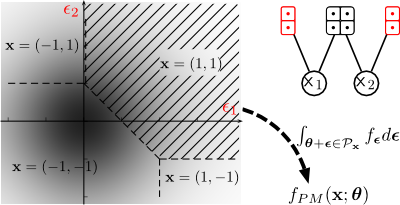A geometric viewpoint is important for understanding discrete-label Perturb-and-MAP random fields. It turns out that the set of perturbations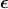for which a particular state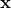will be generated is a polyhedron in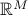. As an illustration, let's consider the Ising model on two variables, with energy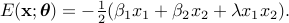Assume perturbations to the unary terms only, i.e.,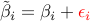. The figure on the left shows its-space geometry. The state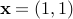, for example, will be generated if the perturbation instance falls in the shaded polyhedron; integrating the perturbation density over this polyhedron gives the probability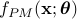of the stateunder the Perturb-and-MAP model.

### Perturbation design: Full and reduced order Gumbel perturbations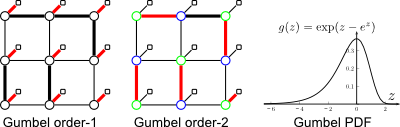While the Perturb-and-MAP and Gibbs models are defined quite differently, it turns out that there exists a particular perturbation design involving the Gumbel probability distribution that makes the two models exactly equivalent. However, this full-order Gumbel perturbation is not very useful in practice as it yields a problem of exponential size. We use instead reduced order perturbations which inject Gumbel noise to unary or pairwise MRF potentials and lead to perturbed energies essentially as easy to minimize as the original unperturbed one. The resulting Perturb-and-MAP model approximates the corresponding Gibbs MRF.

What is the most efficient way to generate in Matlab random samples from a discrete distribution specified in terms of a probability or energy table? Check out this.

### Learning the model parameters from training data by moment matching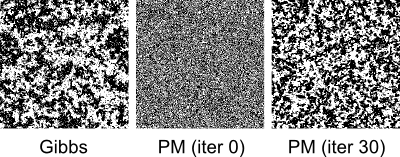We learn model parameters from training data by the moment matching rule, which approximates the maximum likelihood criterion. Similarly to the Gibbs MRF, maximum likelihood estimation in the Perturb-and-MAP model is a concave optimization problem with a unique maximum. The example on the left shows how the moment matching rule learns the parameters of an Ising model from Gibbs Ising model training data. Upon convergence, the generated Perturb-and-MAP samples reproduce the sufficient statistics and also visually closely resemble the training examples.

### Application: Interactive image segmentation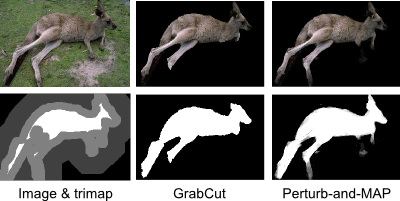Our goal in interactive image segmentation is to find the exact outline of an object given a rough user annotation of the foreground and background. The main advantages of the Perturb-and-MAP model over MAP estimation as used in standard techniques such as GrabCut are: It enables learning the energy model parameters from training data. It produces soft segmentation maps, thus capturing the model's uncertainty on the result. Still, the model still employs graph cuts for sampling and is thus essentially as fast as MAP-based methods.

### Application: Tiered scene labeling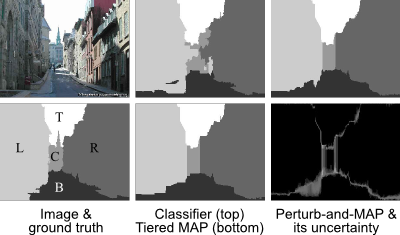As another application, consider the scene labeling problem, where an image of an outdoor scene is segmented into a number of regions (such as facing left, bottom, top, etc.) – see D. Hoiem's geometric context web page for more information. Further, we assume that the scene has a specific tiered structure and also employ an energy function that encourages smoothness in the label assignments – see P. Felzenszwalb's page for further information on the tiered labeling model. Under the Perturb-and-MAP model, we can draw samples by using the efficient tiered dynamic programming MAP algorithm, which enables: Parameter learning from training data. Marginal MAP decisions, which minimize the pixel-wise (Hamming) loss. Uncertainty quantification of the final segmentation result.

## Gaussian Markov Random Fields (NIPS-10 paper)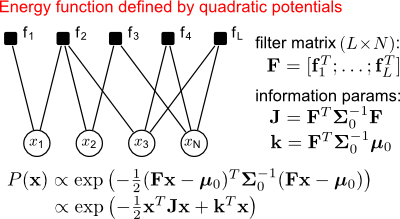In Gaussian models the nodesare real-valued and the energy function is of quadratic form. In Gaussian MRFs each energy factor is typically defined as a quadratic form on the response of a linear filter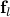with few non-zero coefficients. The support of these filters determine the Markov dependence structure of the model and the sparsity pattern of the inverse covariance (information) matrix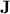. See figure to the left. Gaussian MRFs are popular in spatial statistics and image analysis, both as standalone models and as constituent blocks of more complex non-Gaussian models.

### Exact sampling by local perturbations in Gaussian MRFs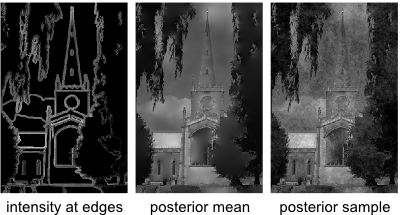The standard approach to sampling from Gaussian models involves computing the Cholesky decomposition of either the covariance or the information matrix. However this is computationally too costly for large scale models such as those encountered in image analysis. We have worked on an alternative scalable method for exact sampling in GMRFs. It amounts to locally perturbing the GMRF potentials by adding Gaussian noise to them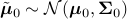, followed by computing the mean/mode of the perturbed GMRF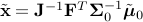. This implies that GMRF sampling has the same computational complexity as GMRF mean computation and allows us to use efficient iterative algorithms such as preconditioned conjugate gradients, loopy Gaussian BP, or DFT-domain techniques for rapid sampling of large-scale GMRFs.

### Variance estimation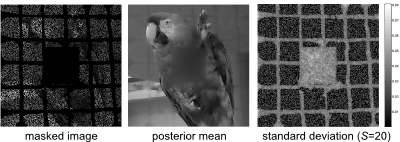The estimation uncertainty in Gaussian MRFs is captured by marginal variances, i.e., the diagonal elements of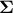. However, computing these variances in large-scale models turns out to be a surprisingly difficult problem. Being able to efficiently draw samples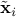from Gaussian MRFs allows us to estimate variances by Monte Carlo,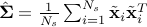, where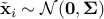. This generic estimator is unbiased and turns out to be very effective, especially when relatively rough variance estimates are required.

### Non-Gaussian continuous MRFs with sparse potentials

Most modern continuous-valued models used in signal and image analysis are non-Gaussian, but instead use ideas from sparse signal modeling. However, Gaussian MRFs turn out to be very useful as building blocks within sparse models. In our work we have shown that efficient Gaussian MRF sampling can be a key ingredient that allows both Monte-Carlo and variational Bayesian approaches scale up to large-scale data.

## Publications

1. G. Papandreou and A. Yuille,
Perturb-and-MAP Random Fields: Reducing Random Sampling to Optimization, with Applications in Computer Vision,
in Advanced Structured Prediction, edited by S. Nowozin, P. Gehler, J. Jancsary, and C. Lampert. MIT Press, 2014.
[pdf] [bib]   (new)
2. G. Papandreou and A. Yuille,
Perturb-and-MAP Random Fields: Using Discrete Optimization to Learn and Sample from Energy Models,
Proc. IEEE Int. Conf. on Computer Vision (ICCV-11), Barcelona, Spain, Nov. 2011.
[pdf] [bib] [appendix] [ICCV talk] [slides] [poster]
3. G. Papandreou and A. Yuille,
Gaussian Sampling by Local Perturbations,
Proc. Int. Conf. on Neural Information Processing Systems (NIPS-10), Vancouver, B.C., Canada, Dec. 2010.
[pdf] [bib] [poster]
4. G. Papandreou, P. Maragos, and A. Kokaram,
Image Inpainting with a Wavelet Domain Hidden Markov Tree Model,
Proc. IEEE Int. Conference on Acoustics, Speech, and Signal Processing (ICASSP-08), pp. 773-776, Las Vegas, NV, U.S.A., Mar.-Apr. 2008.
[pdf] [bib]

## Acknowledgments

Our work has been supported by the U.S. Office of Naval Research under MURI grant N000141010933; the NSF under award 0917141; the AFOSR under grant 9550-08-1-0489; and the Korean Ministry of Education, Science, and Technology, under the National Research Foundation WCU program R31-10008. This is gratefully acknowledged.